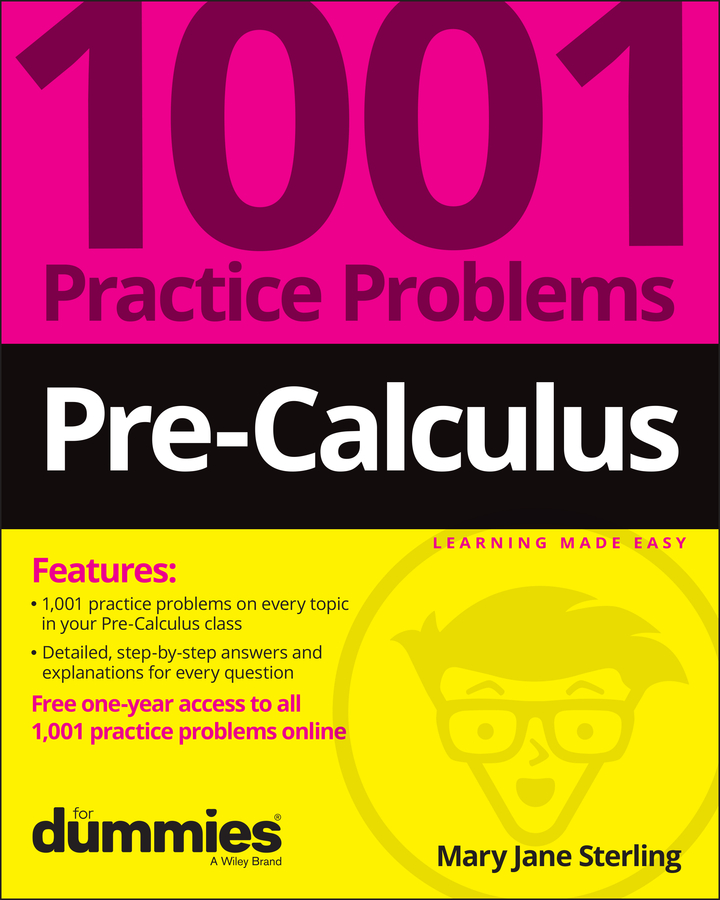##### Pre-Calculus: 1001 Practice Problems For Dummies (+ Free Online Practice)FOIL stands for multiply the first, outside, inside, and last terms together. When you FOIL a binomial times itself, the product is called a perfect square. For example, (a + b)2 gives you the perfect-square trinomial a2 + 2ab + b2. Because a perfect-square trinomial is still a trinomial, you follow the steps in the backward FOIL method of factoring. However, you must account for one extra step at the very end where you express the answer as a binomial squared.

For example, to factor the polynomial 4x2 – 12x + 9, follow these steps:

1. Multiply the quadratic term and the constant term.

The product of the quadratic term 4x2 and the constant 9 is 36x2, so that made your job easy.

2. Write down all the factors of the result that result in pairs in which each term in the pair has an x.

Following are the factors of 36x2 in pairs:

• 1x and 36x

• –1x and –36x

• 2x and 18x

• –2x and –18x

• 3x and 12x

• –3x and –12x

• 4x and 9x

• –4x and –9x

• 6x and 6x

• –6x and –6x

If you think ahead to the next step, you can skip writing out the positive factors, because they produce only x terms with a positive coefficient.

3. From this list, find the pair that adds to produce the coefficient of the linear term.

You want to get a sum of –12x in this case. The only way to do that is to use –6x and –6x.

4. Break up the linear term into two terms, using the terms from Step 3.

You now get 4x2 – 6x – 6x + 9.

5. Group the four terms into two sets of two.

Remember to include the plus sign between the two groups, resulting in (4x2 – 6x) + (–6x + 9).

6. Find the Greatest Common Factor (GCF) for each set and factor it out.

The GCF of the first two terms is 2x, and the GCF of the next two terms is –3; when you factor them out, you get 2x(2x – 3) – 3(2x – 3).

7. Find the GCF of the two new terms.

This time the GCF is (2x – 3); when you factor it out, you get (2x – 3)(2x – 3). Aha! That’s a binomial times itself, which means you have one extra step.

8. Express the resulting product as a binomial squared.

This step is easy: (2x – 3)2.